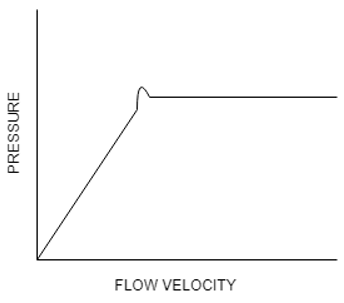Q.1
##### The maximum velocity of the turbulent regime is called the ___________ at which the fast fluidization regime occurs.• a) Terminal velocity
• b) Transport velocity
• c) Superficial velocity
• d) Average velocity
Q.2
##### What happens at velocities above the transport velocity?
• a) Particles coagulate
• b) Particles are carried out of the system by the fluid
• c) Particles break into smaller parts
• d) Particles accumulate at the top
Q.3
##### Which one of the following working model is best suited for fluidized bed combustion boilers?
• a) Bubbling fluidized beds
• b) Laminar film fluidised bed
• c) Pneumatic conveying regime
• d) Moving bed (Dense flow)
Q.4
##### Parameters that describe the overall behaviour of the fluidized bed in the bubbling regime are:
• a) (i) (ii)
• b) (ii) (iii) (iv)
• c) (i) (ii) (iii) (iv)
• d) (i) (ii) (iii)
Q.5
##### Which one of the following method is the most reliable when it comes to determine fluidisation point?
• a) Pressure Drop
• b) Top surface particles starting random movement
• c) Half of the particles getting free
• d) All particles are free
Q.6
##### What is the correct expression for pressure drop in a fluidised bed at fluidisation?
• a) ΔP=(1-∈)(ρP-ρg)gHB
• b) ΔP=(∈)(ρP-ρg)gHB
• c) ΔP=(1-∈)(ρP-ρg)gHC
• d) ΔP=(1-∈)(ρP)gHB
Q.7
##### If the operation on a fluidised bed is a dense flowing fixed bed, then which one of the following method is used for pressure drop calculation?
• a) Sieder Tate Equation
• b) Ergun Equation
• c) DeMentic Equation
• d) Kozeny Carman Equation
Q.8
##### Which one of the heat transfer processes are not involved in a fluidised bed?
• a) Gas-wall heat transfer
• b) Heat transfer between different points in the bed
• c) Heat transfer between the fluidized bed particles and the larger particles floating in the bed
• d) Heat transfer to the submerged surfaces in contact with the bed
Q.9
##### On complete fluidisation, which one of the following is zero?
• a) Gas-particle heat transfer
• b) Heat transfer between different points in the bed
• c) Heat transfer between the fluidized bed particles and the larger particles floating in the bed
• d) Heat transfer to the submerged surfaces in contact with the bed
Q.10
• a) 0.02m2
• b) 0.05m2
• c) 0.04m2
• d) 0.03m2
Q.11
##### Active particles which are quite large sternly repel the emulsion phase and hence the mass transfer rate augments with the ________ of the inert bed material size and _________ with increase of its active particle’s diameter.
• a) Increase, Decrease
• b) Increase, Increase
• c) Decrease, Increase
• d) Decrease, Decrease
Q.12